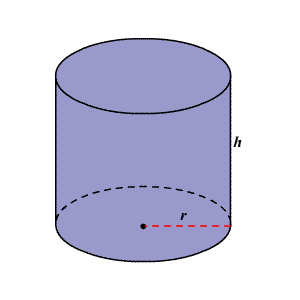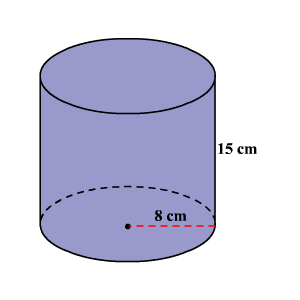# Volume of a Cylinder

A cylinder is a solid composed of two congruent circles in parallel planes, their interiors, and all the line segments parallel to the segment containing the centers of both circles with endpoints on the circular regions.The volume of a $3$ -dimensional solid is the amount of space it occupies.  Volume is measured in cubic units ( ${\text{in}}^{3},{\text{ft}}^{3},{\text{cm}}^{3},{\text{m}}^{3}$ , et cetera).  Be sure that all of the measurements are in the same unit before computing the volume.

The volume $V$ of a cylinder with radius $r$ is the area of the base $B$ times the height $h$ .

$V=Bh\text{\hspace{0.17em}}\text{\hspace{0.17em}}\text{or}\text{\hspace{0.17em}}\text{\hspace{0.17em}}V=\pi {r}^{2}h$

Example:

Find the volume of the cylinder shown. Round to the neatest cubic centimeter.Solution

The formula for the volume of a cylinder is $V=Bh\text{\hspace{0.17em}}\text{\hspace{0.17em}}\text{or}\text{\hspace{0.17em}}\text{\hspace{0.17em}}V=\pi {r}^{2}h$ .

The radius of the cylinder is $8$ cm and the height is $15$ cm.

Substitute $8$ for $r$ and $15$ for $h$ in the formula $V=\pi {r}^{2}h$ .

$V=\pi {\left(8\right)}^{2}\left(15\right)$

Simplify.

$\begin{array}{l}V=\pi \left(64\right)\left(15\right)\\ \approx 3016\end{array}$

Therefore, the volume of the cylinder is about $3016$ cubic centimeters.